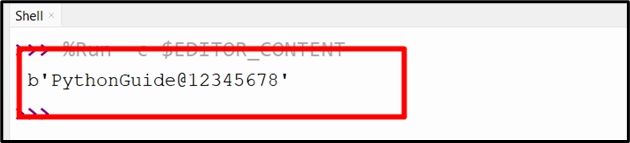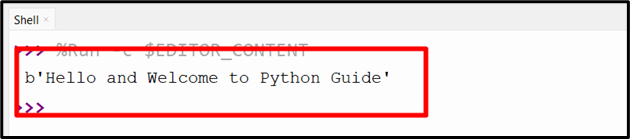| by Arround The Web | No comments

## Python Gzip Decompress Function

Data compression is a crucial method that aids in decreasing the volume of data, making it more manageable for purposes of storage and transmission. Python’s “gzip” module offers a simple approach to compress and decompress files utilizing the “GZIP” algorithm. The “decompress()” function of the “gzip” module is used to decompress the data that has been compressed via the GZIP algorithm.

In this article, we will explore the “gzip.decompress()” function in detail using numerous examples.

## What is GZIP?

GZIP is a “file format” and software application utilized for compressing and decompressing files.

It relies on the DEFLATE algorithm, which is a fusion of LZ77 and Huffman coding, “GZIP” compression works by analyzing the data to be compressed and creating a set of Huffman codes that represent the most frequently occurring data patterns. These codes are used to encode the data, resulting in a compressed file that is smaller in size than the original data.

GZIP compression is utilized to decrease the size of files for storage and transmission purposes. It is useful especially when dealing with large files, such as multimedia files or data sets.

## Python “gzip.decompress()” Function

The “gzip.decompress()” function is used to decompress the data that has been compressed via the “GZIP” algorithm. This function takes a compressed data string as an argument and returns the original uncompressed data.

Syntax

gzip.decompress(data, wbits=MAX_WBITS, bufsize=DEFAULT_BUFFER_SIZE)

In the above syntax, the “gzip.decompress()” function takes the following parameters:

• The “data” parameter is a string that represents the compressed data requiring decompression.
• The “wbits” parameter refers to an integer specifying the number of bits to use for the window size. The default value is “MAX_WBITS”, which corresponds to a “32” KB window size.
• The “bufsize” corresponds to an integer specifying the size of the buffer used for decompression. The default value is “DEFAULT_BUFFER_SIZE”, which corresponds to “16” KB.

Let’s understand it via the following example:

Example 1: Decompress GZIP Compressed String
Here’s an example of using the “gzip.decompress()” function to remove/decompress GZIP compression:

import gzip
data = b'Hello and Welcome to Python Guide'
data = gzip.compress(data)
print(gzip.decompress(data))

In the above lines of code:

• The “gzip” module is imported and a variable representing the GZIP compressed data string is initialized.
• The “gzip.decompress()” function takes the compressed data string as an argument and returns the original uncompressed data as a byte string.

OutputHere, the byte string has been created from the compressed data string.

Example 2: Decompress the Compressed GZIP Byte Object
Let’s overview the following example code:

import gzip
data = b'PythonGuide@12345678'
data = gzip.compress(data)
print(gzip.decompress(data))

In the above code:

• The “gzip” module is imported and the input byte data is initialized in the program.
• The “gzip.compress()” function is applied to compress the bytes object.
• Lastly, the “gzip.decompress()” function is used to decompress the compressed data.

OutputIn the above output, the byte string has been created from the compressed data string.

Example 3: Decompress the Compressed GZIP File
Consider the following example:

import gzip
with gzip.open('file_1.gz', 'rb') as f:
print(decompressed_data)

In the above code snippet:

• The “gzip” module is imported.
• After that, the “gzip.open()” function is used to open a file in binary mode with the ‘rb’ flag.
• The contents of this file are then read using the “read()” method.
• The “gzip.decompress()” function of the gzip module is utilized to decompress the data.

OutputIn the above output, the gzip compressed file has been decompressed via the “gzip.decompress()” method.

## Conclusion

The “gzip.decompress()” function is a powerful tool for decompressing data that has been compressed via the “GZIP” algorithm. It is an essential function for any Python developer to work with compressed data files. This function takes a compressed data string as an argument and returns the original uncompressed data. This Python post provided a thorough guide on the “gzip.decompress()” function using numerous examples.

Source: linuxhint.com